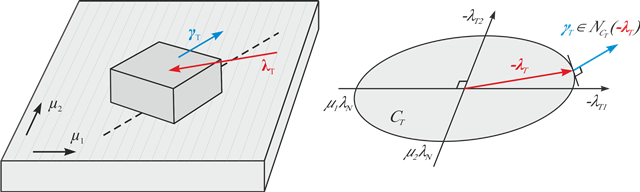# Anisotropic dry friction

The aim of this project is to establish a valid description of anisotropic dry friction by analyzing the set of admissible friction forces and investigating the relationship between the sliding direction and the friction force.

The magnitude and direction of friction forces acting on a body moving over a machined surface are often found to vary with the direction of sliding. Machining or finishing of a surface can lead to an anisotropic surface roughness which in turn causes anisotropic friction. In addition, anisotropy can originate from the crystal structure of a material and occurs on the surface of composite, textile and biological materials. The classical Coulomb friction law describes the constitutive behavior of stick and slip resulting in a set-valued force law. In the framework of convex analysis, the set-valued force law can be expressed as the subdifferential of a convex pseudo potential, i.e. the dissipation function. Furthermore, the contact law for pure Coulomb friction states that the vector of the sliding velocity lies within the normal cone of the friction force on a closed convex set of admissible contact forces. For isotropic friction, the set of admissible forces or force reservoir is given by a circular friction disk. Therefore, in the isotropic case, the friction force is always co-linear to the direction of the sliding velocity and opposes the direction of sliding. For anisotropic friction, however, the friction force is found to be no longer co-linear to the sliding direction. The generalization of the contact law to anisotropic friction by taking an arbitrary closed convex force reservoir and assuming the principle of maximal dissipation to hold, leads again to a normal cone inclusion force law.

So far, the validity of the force law for anisotropic friction has not been proven. In this project, the normal cone inclusion force law for anisotropic friction is investigated experimentally by measuring the components of the friction force for varying sliding paths and the shape of the force reservoir is analyzed. Ultimately, a mathematical description is to be found that allows for an accurate prediction of the behavior of a body undergoing anisotropic friction.Body sliding over anisotropic surface (left) and sliding velocity represented as normal cone inclusion on closed convex friction force reservoir (right).

Publications

Walker, S.V. and Leine, R.I.: "Set-valued anisotropic dry friction laws: formulation, experimental verification and instability phenomenon", in  Nonlinear DynamicsVol. 96, pp. 885–9202019. PDF, Link

Walker, S. and Leine, R.I.: "Anisotropic dry friction with non-convex force reservoirs: modeling and experiments", Proc. ENOC, Budapest, Hungary, 2017. PDF

Walker, S.V. and Leine, R.I.: "Modeling and numerical simulation of anisotropic dry friction with non-convex friction force reservoir", Proc. IMSD, Montréal, Canada, 2016. PDF

### Kontakt### Remco I. Leine

Prof. Dr. ir. habil.

Direktor